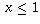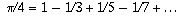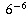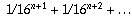Navigation Panel:(These buttons explained below)Question Corner and Discussion Area

# Calculating Digits of Pi in Other Bases

Asked by an anonymous poster on December 12, 1997:
Could you please give us a formula for figuring out what pi is in base 6? We have the numbers 3,0,5,0,3,3,0,0,5,1,4,5, etc. but we do not know exactly how to obtain these numbers.
Digits of pi are generally calculated by representing pi as the sum of an infinite series, then evaluating that sum to as many terms as are necessary to get the desired number of digits.

One way is to use the formula Arctan(x) = x - x^3 + x^5/5 - x^7/7 + . . . , which is valid for. Plugging in x=1 is not much use because, although you get a formula, the series converges very slowly and you have to take thousands of terms to get even a few digits of accuracy.

A faster way is to use a formula such as. You evaluate each of these arctangents using the above series, taking enough terms of the series to get you to within the accuracy you desire. In your case, you would do the arithmetic in base 6 to get digits ofin base 6.

Another, more recent, series iswhich has the advantage that in base 16 there turns out to be a clever way to use this formula to calculate far-out digits ofwithout having to do high-precision arithmetic and without having to compute all the earlier digits first. That's only a help in base 16 and a few other bases, though.

For example, if you were to use this latter series to calculate the first five base-6 digits of, you would say that you wantto within an accuracy of(which is about 0.00002). Note that the k-th term in the above series is smaller than, so if you add up the terms from k=n+1 onwards the most you will get iswhich, by the formula for summing a geometric series, is. This, then, is the maximum error you could possibly introduce by taking only the first n terms of the series.

If you take n=3, the above estimate gives you an error of at most 0.0000163 which is smaller than your desired accuracy. Therefore, all you need to do is take the first three terms of the series. You would evaluate the terms when k=0, when k=1, when k=2, and when k=3 and add them up, doing the calculations in base 6.

[ Submit Your Own Question ] [ Create a Discussion Topic ]

This part of the site maintained by (No Current Maintainers)
Last updated: April 19, 1999
Original Web Site Creator / Mathematical Content Developer: Philip Spencer
Current Network Coordinator and Contact Person: Joel Chan - mathnet@math.toronto.edu

Navigation Panel: